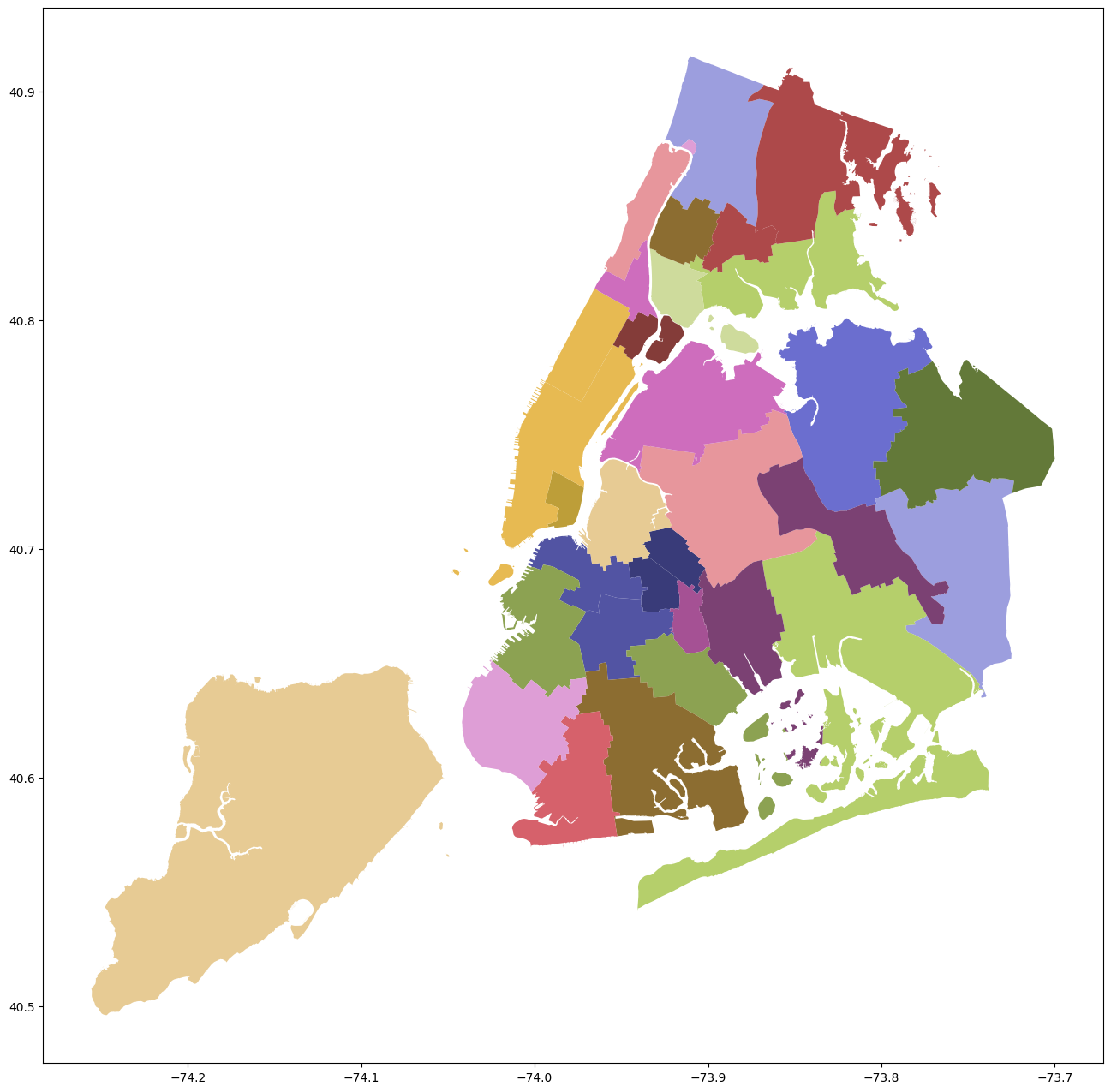# Introduction to Maps and Spatial Data#

These examples use the GeoPandas package which adds special functions for DataFrames to work with geographic data.

There are two basic ways to visualize the data spatial data (aka make maps): plot() and explore(). plot() creates a matplotlib chart of the data, explore() creates an interactive map with the shapes overlayed on open map data.

For the visualizations to work, though, you have to set a relevant index on the data. In our case here, the index is the district number.

After the basic plots, we merge the school district data with the school demographics data in order to create more detailed examples.

# uncomment and run if using Google Colab

# !pip install geopandas
# !pip install nycschools

import pandas as pd
import geopandas as gpd
import numpy as np
import matplotlib.pyplot as plt
import seaborn as sns
from IPython.display import Markdown as md

from nycschools import schools, geo, ui


## GeoDataFrame#

GeoDataFrame adds geospatial data to a regular pandas DataFrame. The specially named geometry column is used to plot the spatial data on a map.

# read the GeoJSON file directly from the download link
# each shape in "geometry" represents a district
gdf = gdf.set_index("district")

area length geometry
district
32 51898496.7618 37251.0574964 MULTIPOLYGON (((-73.91181 40.70343, -73.91290 ...
16 46763620.3794 35848.9043428 MULTIPOLYGON (((-73.93312 40.69579, -73.93237 ...
17 128440514.645 68356.1032412 MULTIPOLYGON (((-73.92044 40.66563, -73.92061 ...
13 104871082.804 86649.0984086 MULTIPOLYGON (((-73.97906 40.70595, -73.97924 ...
25 443759165.29 176211.272136 MULTIPOLYGON (((-73.82050 40.80101, -73.82040 ...
# draw the basic map using the tab20b color map
_ = gdf.plot(figsize=(16, 16), cmap="tab20b")# explore gives us an interactive map
# non-geo columns show up when you hover over a shape
gdf.explore()

Make this Notebook Trusted to load map: File -> Trust Notebook